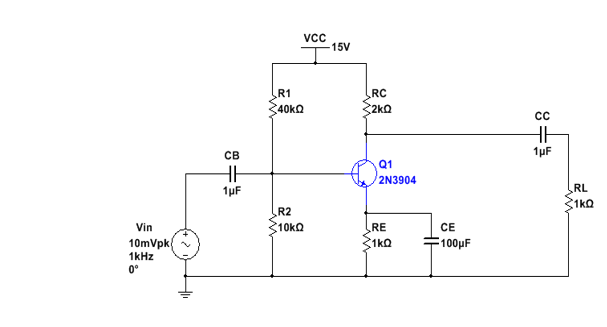# Week 6 Lab Solved

25.00 \$

Category:

## Description

• Part A:
1. Analyze the circuit or the amplifier shown in Figure 1. Determine the voltages VB, VE, VC, VBE and VCE. Using the beta value of 100, determine the gain of the amplifier (AV). (Include 20% tolerances for resistors and capacitors).
2. Construct the circuit shown in Figure 1 on the breadboard using the hardware parts.
3. Using the jumper wires, screw driver and screw terminal connector, connect the board to NI MyDAQ Instrument Device.
4. Using the function generator in NI ELVISmx Instrument Launcher, provide the voltage (Vin). Use channel AO0 pin on the NI myDAQ Instrument Device to provide this voltage. Make sure the frequency is 1 KHz frequency.
5. Using +15V pin out on the Instrument Device provide the supply voltage VCC.
6. Perform the DC Analysis by disconnecting the capacitors and measuring VB, VE, VC, IB, IE and IC.
7. Place the capacitors back and using pin AI0 on the NI myDAQ Instrument Device, measure the required voltages: VB, VE, VC VBE, VCE.
8. Measure the currents: IC and IE using the Multimeter.
9. Use AI1 on the NI myDAQ Instrument Device to measure the load voltage VRL. Record the measurements and compare them with the calculated values in step 1.
10. Using the input voltage Vin, base voltage VB and load voltage VRL, determine the gain of the amplifier (AV).

Figure 1